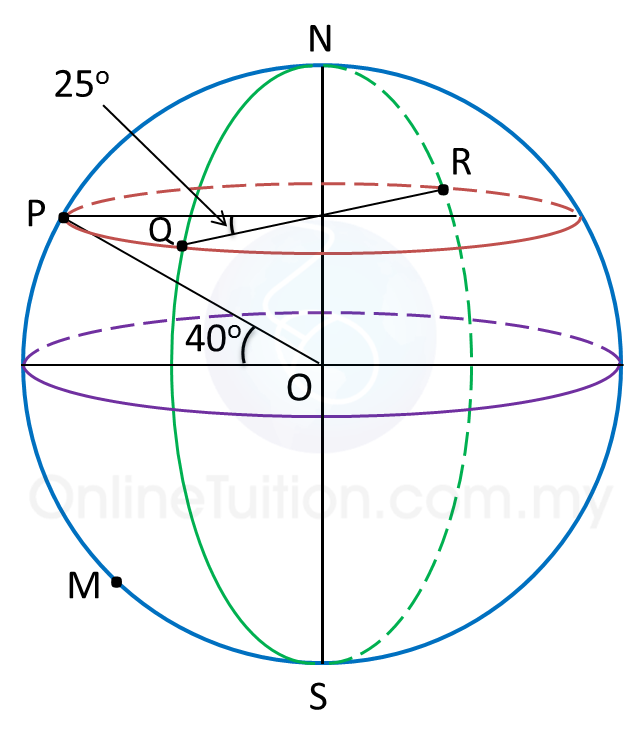# 9.6 Earth as a Sphere, SPM Paper 2 (Long Questions)

Question 1:
Diagram below shows four points P, Q, R and M, on the surface of the earth. lies on longitude of 70oW. QR is the diameter of the parallel of latitude of 40o N. M lies 5700 nautical miles due south of P.(a) Find the position of R.
(b) Calculate the shortest distance, in nautical miles, from Q to R, measured along the surface of the earth.
(c) Find the latitude of M.
(d) An aeroplane took off from R and flew due west to P along the parallel of latitude with an average speed of 660 knots.
Calculate the time, in hours, taken for the flight.

Solution:
(a)
Latitude of = latitude of Q = 40o N
Longitude of = (70o – 25o) W = 45o W
Longitude of = (180o – 45o) E = 135o E
Therefore, position of R = (40o N, 135oE).

(b)
Shortest distance from Q to R
= (180 – 40 – 40) x 60
= 100 × 60
= 6000 nautical miles

(c)
$\begin{array}{l}\angle POM=\frac{5700}{60}\\ \text{}={95}^{o}\\ \therefore \text{Latitude of}M=\left({95}^{o}-{40}^{o}\right)S\\ \text{}={55}^{o}S\end{array}$

(d)
$\begin{array}{l}\text{Time taken =}\frac{\text{distance from}R\text{to}P}{\text{average speed}}\\ \text{}=\frac{\left(180-25\right)×60×\mathrm{cos}{40}^{o}}{660}\\ \text{}=\frac{155×60×\mathrm{cos}{40}^{o}}{660}\\ \text{}=10.79\text{hours}\end{array}$

Question 2:
P (25o N, 35o E), Q (25o N, 40o W), R and S are four points on the surface of the earth. PS is the diameter of the common parallel of latitude 25o N.
(a) Find the longitude of S.
(b) R lies 3300 nautical miles due south of P measured along the surface of the earth.
Calculate the latitude of R.
(c) Calculate the shortest distance, in nautical mile, from P to S measured along the surface of the earth.
(d) An aeroplane took off from R and flew due north to P. Then, it flew due west to Q.
The total time taken for the whole flight was 12 hours 24 minutes.
(i) Calculate the distance, in nautical mile, from P due west Q measured along the common parallel of latitude.
(ii) Calculate the average speed, in knot, of the whole flight.

Solution:
(a)
Longitude of S = (180o – 35o) W = 145o W

(b)

(c)
Shortest distance from P to S
= (65 + 65) x 60
= 130 x 60
= 7800 nautical miles

(d)(i)
Distance of PQ
= (35 + 40) x 60 x cos 25o
= 75 x 60 x cos 25o
= 4078.4 nautical miles